# Quadrant Scatter Chart in Excel

## Quadrant & scatter diagrams compare the relationship between two variables

The difference is the placement of the Y axis in relationship to the X axis.
Both of these charts are easy to create using QI Macros add-in for Excel.

### Scatter Diagram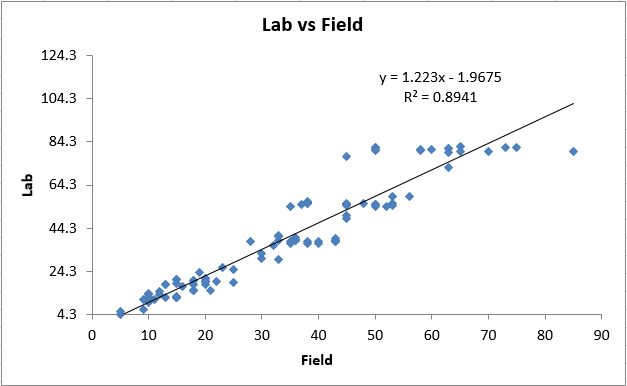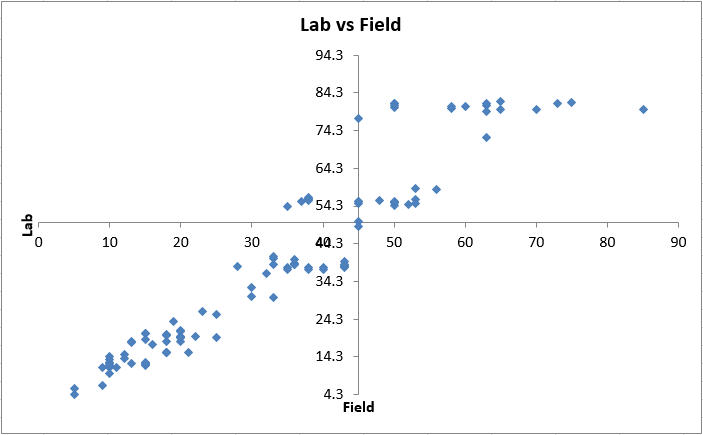Then, select "Box, Dot & Scatter Plot" > Quadrant ScatterNext, you will be asked to input the point at which the Y-Axis crosses the X-Axis and the X-Axis Crosses the Y-Axis - this creates your quadrant. Note that we also identify potential crossings for you: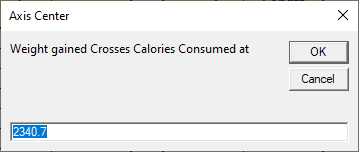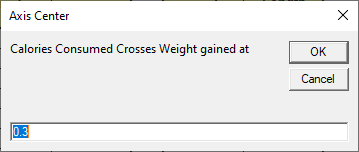A Quadrant Scatter Chart will then be created:### You can also manually convert a scatter plot to a four-quadrant graph

1. First, delete the trend line from your scatter diagram.Right click on the X-axis, select Format Axis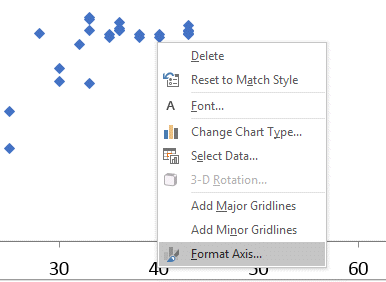Now change the value where you want the vertical Y-axis to cross the X-axis. In this example, we chose 45.Right click on the Y-axis, select Format AxisNow change the value where you want the horizontal X-axis to cross the Y-axis. In this example, we chose 50.4. Once you have updated both the X and Y Axis Values, you should have a XY Scatter Diagram with Four Quadrants!### Other Charts Included in QI Macros Excel add-in

Control Charts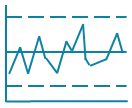Histograms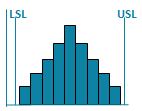Pareto ChartsBox PlotsFishbone Diagram